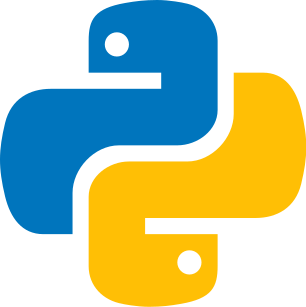Last update: May 16th, 2023

Data structures allow to organize, manage and store data in memory. We can modify them and access these data thanks to methods (functions). There are several data structures; we will see them in several parts. We start with arrays.### Definition of an array

Arrays store values of the same type or different types depending on the programming language. The position of each element is named index and starts with 0 and not with 1. It is crucial to consider this element, for example, when using a for loop. Also, you can create an empty array and then fill it later using methods. This is called initialization.

Here the arrays are represented by a train. Indeed, a train can carry (contain) several elements, like an array. Likewise, a train can be empty, just like an array.

#### Codepython
1empty = []
2print(empty)  # []
3
4fruits = ['apple', 'lemon']
5print(fruits)  # [ 'apple', 'lemon' ]

### Content of an array

Arrays can be composed of real (integers, decimals), character strings, and depending on the programming languages, literal objects, or even a mixture of different types (for example, JavaScript or Python).

Trains can contain any type of passenger, but there is a certain limit. It is the case of the arrays, which can contain numbers and letters in a general way.

#### Codepython
1numbers = [1, 3, 5, 6]
2print(numbers)  # [ 1, 3, 5, 6 ]
3
4fruits = ['apple', 'lemon']
5print(fruits)  # [ 'apple', 'lemon' ]
6
7mix = [1, 'apple', 3, True, {'color': 'blue'}]
8print(mix)  # [ 1, 'apple', 3, true, { color: 'blue' } ]

To access an element, we use the indices, i.e., the position of each element. We count from zero. We use two brackets to access it.

In a train, each seat has a number that corresponds to the position of the seat. This corresponds to the position of each element of an array. Here we start the seats at zero as in an array.

#### Codepython
1fruits = ['apple', 'lemon']
2mix = [1, 'apple', 3, True, {'color': 'blue'}]
3
4print(fruits)  # apple
5print(mix)  # 3

### Methods for an array

To manipulate arrays, we will use what we call methods. These are functions specific to each type. For example, if we want to know the length of an array (how many elements in total), we will use the method .length. There are many methods.

You can count the number of wagons in a train, which corresponds to the length of an array. For example, a train with ten seats will correspond to an array of ten indices. You can also remove wagons from the train, as you can remove indices from an array.

#### Codepython
1numbers = [1, 3, 5, 6]
2mix = [1, 'apple', 3, True, {'color': 'blue'}]
3
4print(mix)  # [1, 'apple', 3, True, {'color': 'blue'}]
5
6print(numbers[2:])  # [5, 6]
7numbers.append(1000)
8print(numbers)  # [1, 3, 5, 6, 1000]
9
10print(len(numbers))  # 5

### Multidimensional arrays

Arrays can be nested so that they are multidimensional. To access the keys, we will proceed by order: the first bracket will correspond to the first array, the second bracket to the second, and so on. If we want to access the index of a child array, we will add a second bracket.

#### Codepython
1multi_array = [[1, 2, 3, 4], [5, 6, 7]]
2
3print(multi_array)  # [ 1, 2, 3, 4 ]
4print(multi_array)  # 3Arrays are data structures that are widely used in programming. They allow the assembling of several elements at the same time. Methods can manipulate them. Elements are accessed through indices that start with 0. You can also create arrays with several dimensions.